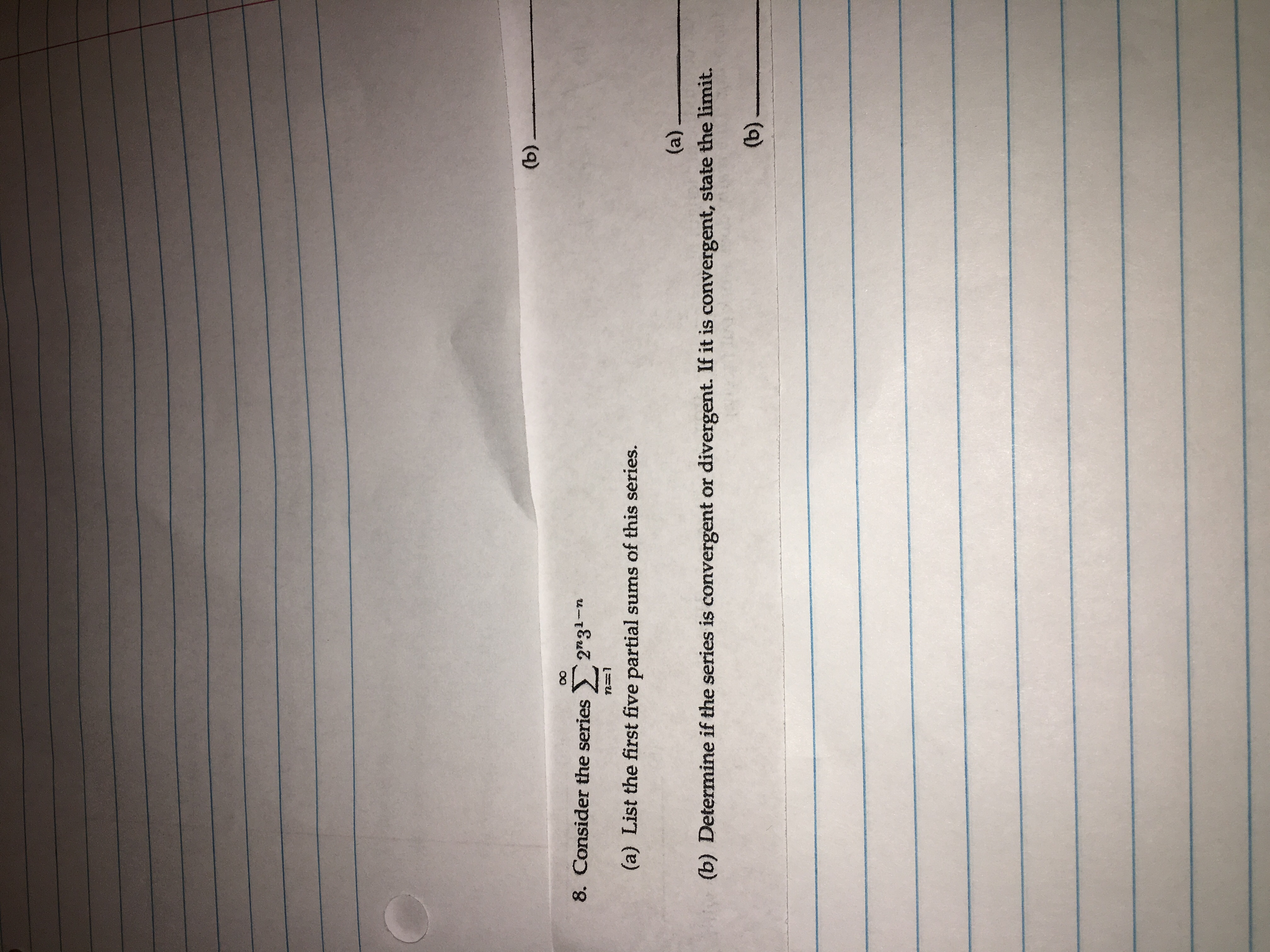# 8. Consider the series22 31-n(a) List the first five partial sums of this series.(b) Determine if the series is convergent or divergent. If it is convergent, state the limit.(b)一一

Question
1 viewshelp_outlineImage Transcriptionclose8. Consider the series 22 31-n (a) List the first five partial sums of this series. (b) Determine if the series is convergent or divergent. If it is convergent, state the limit. (b)一一 fullscreen
check_circle

Step 1

To calculate the required partial sums and discuss the convergence of the series

Step 2

The first five partial sums are 2, 3.333.....

### Want to see the full answer?

See Solution

#### Want to see this answer and more?

Solutions are written by subject experts who are available 24/7. Questions are typically answered within 1 hour.*

See Solution
*Response times may vary by subject and question.
Tagged in

### Other